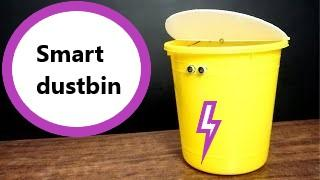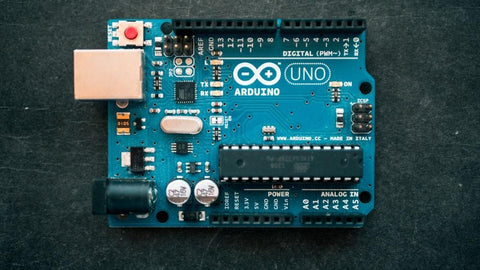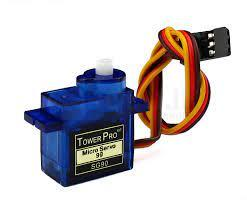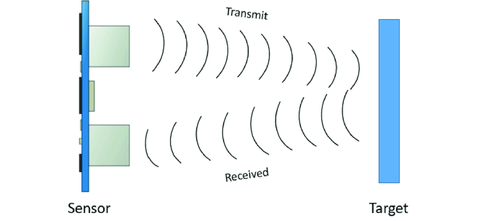# Smart Dustbin using Arduino

## Introduction

In this article, we will be looking at how to make a smart dustbin using Arduino, an ultrasonic sensor, and an SG-90 servo motor. Let's start!## Hardware Required

We will need the following components for making the smart dustbin

If you don't have any of the hardware then you can click on the required component name and buy it from our store.

### 1. Arduino UnoIt is a development board used to do all the processing.

2. Ultrasonic Sensor HC-SR-04

It is used as an object detection sensor. It is used to measure the distance of the object from the sensor.### 3. SG-90 Servo

A Servo motor is used for moving the dust bin lid.### 4. Jumper Wires

Used to do circuit connections between different components.## Working Principle

Smart Dustbin works on the principle of object detection using an ultrasonic sensor.  The ultrasonic sensor transmits sound waves. These waves get reflected whenever an object comes into the vicinity of the sensor. This generates an electrical signal which is used to open the dustbin lid.## Circuit Diagram

Make the connections as shown in the diagram below.## Program Code

 /*  SMART DUSTBIN ARDUINO CODE  Lid of the dustbin gets opened when a person comes in range of 30cm in front of the dustbin*/#include   //INCLUDES SERVO LIBRARYServo servo;int trigPin = 5;int echoPin = 6;int servoPin = 9;long duration, distance, average;long averDist;void setup() {  Serial.begin(9600);  servo.attach(servoPin);  pinMode(trigPin, OUTPUT);  pinMode(echoPin, INPUT);  servo.write(0); //CLOSES CAP ON STARTING  delay(100);  servo.detach();}void measure() {  digitalWrite(trigPin, LOW);  delayMicroseconds(5);  digitalWrite(trigPin, HIGH);  delayMicroseconds(15);  digitalWrite(trigPin, LOW);  pinMode(echoPin, INPUT);  duration = pulseIn(echoPin, HIGH);  distance = (duration / 2) / 29.1; //CALCULATES DISTANCE}void loop() {  Serial.println(distance);      for (int i = 0; i <= 2; i++) { //CALCULATES AVERAGE DISTANCE    measure();    averDist[i] = distance;    delay(10);  }  distance = (averDist + averDist + averDist) / 3;  if ( distance <= 30 ) {    servo.attach(servoPin);    delay(1);    servo.write(180);    delay(3500);  }  else    servo.write(0);    delay(1500);    servo.detach(); }

Servo servo;

This creates servo object.

 int trigPin = 5;int echoPin = 6;int servoPin = 9;

The above lines assign trigger and echo pins of the ultrasonic sensor to 5 and 6 respectively. Servo’s PWM pin is connected to pin 9 of Arduino.

long duration, distance, average;
long averDist;

These are the variables created for storing and processing data from ultrasonic sensor.

 servo.attach(servoPin);  pinMode(trigPin, OUTPUT);  pinMode(echoPin, INPUT); servo.write(0); //CLOSES CAP ON STARTING  delay(100);  servo.detach();

servo. Attach (servoPin) - attaches the servo variable which we created initially to the servo pin, set trigger and echo pins as Output and Input respectively, close the lid of the dustbin by setting servo to 0 positions, and then detach the servo object.

 Serial.println(distance);      for (int i = 0; i <= 2; i++) { //CALCULATES AVERAGE DISTANCE    measure();    averDist[i] = distance;    delay(10);  }  distance = (averDist + averDist + averDist) / 3;

Measure function calculates the distance and average calculates the average of 3 distances measured by ultrasonic sensor.

 if ( distance <= 30 ) {    servo.attach(servoPin);    delay(1);    servo.write(180);    delay(3500);  }  else    servo.write(0);    delay(1500);    servo.detach();

If the distance is less than 30 then the dustbin lid is opened by setting the servo to 180 position otherwise the dustbin’s lid is closed.

## Conclusion

In this blog, we made a smart dustbin that automatically opens and closes based on the presence of the person in the vicinity.

Feel free to comment if you have questions!